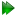Subsections

Model-free optimisation theory

The implementation of optimisation in relax is discussed in detail in Chapter 14. To understand the concepts in this subsection, it is best to look at that chapter first.

The model-free space

In model-free analysis the target function f (θ) is the chi-squared equation

 χ2(θ) =, (7.23)

where i is the summation index, Ri is the experimental relaxation data which belongs to the data set R and includes the R1, R2, and NOE values at all field strengths, Ri(θ) is the back calculated relaxation data belonging to the set R(θ), and σi is the experimental error. For the optimisation of the model-free parameters while the diffusion tensor is held fixed, the summation index ranges over the relaxation data of an individual spin. If the diffusion parameters are optimised simultaneously with the model-free parameters the summation index ranges over all relaxation data of all selected spins of the macromolecule.

Given the current parameter values the model-free function provided to the algorithm will calculate the value of the model-free spectral density function J(ω) at the five frequencies which induce NMR relaxation by using Equations (7.7) and (7.8). The theoretical R1, R2, and NOE values are then back-calculated using Equations (7.3a), (7.3b), (7.3c), and (7.6). Finally, the chi-squared value is calculated using Equation (7.24).

To produce the gradient and Hessian required for model-free optimisation a large chain of first and second partial derivatives needs to be calculated. Firstly the partial derivatives of the spectral density functions (7.7) and (7.8) are necessary. Then the partial derivatives of the relaxation equations (7.3a) to (7.3c) followed by the NOE equation (7.6) are needed. Finally the partial derivative of the chi-squared formula (7.24) is required. These first and second partial derivatives, as well as those of the components of the Brownian diffusion correlation function for non-isotropic tumbling, are presented as Chapter 15.

Grid search

Due to the complexity of the curvature of the model-free space, the grid point with the lowest chi-squared value may in fact be on the opposite side of the space to the local minimum. Therefore the model-free space renders many optimisation algorithms ineffective (d'Auvergne and Gooley, 2008b).

Parameter constraints

To understand this section, please see Section 14.5 on page. For model-free analysis, linear constraints are the most useful type of constraint as the correlation time τf can be restricted to being less than τs by using the inequality τs - τf0.

For the parameters specific to individual spins the linear constraints in the notation of (14.18) are⋅. (7.24)

Through the isolation of each individual element, the constraints can be seen to be equivalent toTo prevent the computationally expensive optimisation of failed models in which the internal correlation times minimise to infinity (d'Auvergne and Gooley, 2006), the constraint τe, τf, τs2τm was implemented. When the global correlation time is fixed the constraints in the matrix notation of (14.18) are⋅. (7.26)

However when the global correlation time τm is one of the parameters being optimised the constraints become⋅. (7.27)

For the parameters of the diffusion tensor the constraints utilised arewhich in the matrix notation of (14.18) become⋅. (7.29)

The upper limit of 200 ns on τm prevents the parameter from heading towards infinity when model failure occurs (see d'Auvergne and Gooley (2006)). This can significantly decrease the computation time. To isolate the prolate spheroid the constraint⋅, (7.30)

is used whereas to isolate the oblate spheroid the constraint used is⋅. (7.31)

Dependent on the model optimised, the matrix A and vector b are constructed from combinations of the above linear constraints.

Diagonal scaling

The concept of diagonal scaling is explained in Section 14.6 on page.

For the model-free analysis the scaling factor of one is used for the order parameter and a scaling factor of 1e-12 is used for the correlation times. The Rex parameter is scaled to be the chemical exchange rate of the first field strength. The scaling matrix for the parameters {S2, S2f, S2s, τe, τf, τs, Rex, r, CSA} of individual spins is. (7.32)

For the ellipsoidal diffusion parameters {τm,,, α, β, γ} the scaling matrix is. (7.33)

For the spheroidal diffusion parameters {τm,, θ, φ} the scaling matrix is. (7.34)

The relax user manual (PDF), created 2020-08-26.